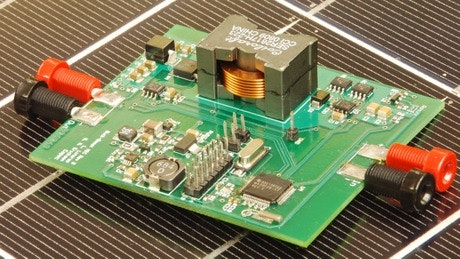>>

## Introduction to Power Electronics

### University/Institute: University of Colorado Boulder## Description

This course can also be taken for academic credit as ECEA 5700, part of CU Boulder's Master of Science in Electrical Engineering degree. This course introduces the basic concepts of switched-mode converter circuits for controlling and converting electrical power with high efficiency. Principles of converter circuit analysis are introduced, and are developed for finding the steady state voltages, current, and efficiency of power converters. Assignments include simulation of a dc-dc converter, analysis of an inverting dc-dc converter, and modeling and efficiency analysis of an electric vehicle system and of a USB power regulator. After completing this course, you will: ● Understand what a switched-mode converter is and its basic operating principles ● Be able to solve for the steady-state voltages and currents of step-down, step-up, inverting, and other power converters ● Know how to derive an averaged equivalent circuit model and solve for the converter efficiency A basic understanding of electrical circuit analysis is an assumed prerequisite for this course.